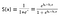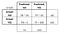# Machine Learning Model: Logistic RegressionThe formula for the sigmoid function. The function has a value between 0 and 1, which gives you the probability.
`from sklearn.linear_model import LogisticRegression`
`classifier = LogisticRegression()`
`classifier.fit(X_train, y_train)`
`y_pred = classifier.predict(X_test)`An example of a confusion matrix. In the diagram, the number of incorrect predictions are 5 and 10, while the number of correct predictions are 50 and 100. Do you see how?
`from sklearn.metrics import confusion_matrix`
`cm = confusion_matrix(y_test, y_pred)`

“Visuals allows us to better understand insights and allow communicate these insights to other people. The data is often displayed in a story format that visualizes patterns, trends and correlations that may otherwise go unnoticed.”

## Data Driven Investor

### By Data Driven Investor

In each issue we share the best stories from the Data-Driven Investor's expert community. Take a look

Written by

Written by

## Amitabha Dey